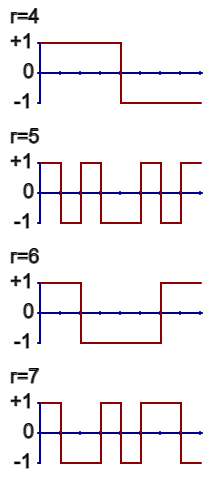By Sean O’Connor, a science and technology author and investigator.

The fast Walsh Hadamard transform (WHT) is a simplified version of the Fast Fourier Transform (FFT.)

The 2-point WHT of the sequence a, b is just the sum and difference of the 2 values:

It is self-inverse allowing for a fixed constant:

Due to (a+b) + (a-b) = 2a and (a+b) – (a-b) = 2b.

The constant can be split between the two Walsh Hadamard transforms using a scaling factor of √2 to give a normalized WHTN:

```WHTN(a, b) = (a+b)/√2, (a-b)/√2

WHTN((a+b)/√2, (a-b)/√2) = a, b

```

That particular constant results in the vector length of a, b being unchanged after transformation since a2+b2 =((a+b)/√2)2+ ((a-b)/√2)2 as you may easily calculate.

The 2-point transform can be extended to longer sequences by sequentially adding and subtracting pairs of similar terms, alike in the pattern of + and – symbols they contain.

To transform a 4-point sequence a, b, c, d first do two 2-point transforms:

```WHT(a, b) = a+b, a-b

WHT(c, d) = c+d, c-d

```

Then add and subtract the alike terms a+b and c+d:

```WHT(a+b, c+d) = a+b+c+d, a+b-c-d

```

and the alike terms a-b and c-d:

```WHT(a-b, c-d) = a-b+c-d, a-b-c+d

```

The 4-point transform of a, b, c, d then is

```WHT(a, b, c, d) = a+b+c+d,  a+b-c-d, a-b+c-d, a-b-c+d

```

When there are no more similar terms to add and subtract, that signals completion (after log2(n) stages, where n is 4 in this case.)  The computational cost of the algorithm is nlog2(n) add/subtract operations, where n, the size of the transform, is restricted to being a positive integer power of 2 in the general case.

If the transform was done using matrix operations, the cost would be much higher (n2 fused multiply-add operations.)

Figure 1.  The 4-point Walsh Hadamard transform calculated in matrix form.

The +1, -1 entries in Figure 1 are presented in a certain natural order which most of the actual algorithms for calculating the WHT result in, which is fortunate since then the matrix is symmetric, orthogonal and self-inverse.

You can also view the +1, -1 patterns of the WHT as waveforms.Figure 2.  The waveforms of the 8-point WHT presented in natural order.

When you calculate the WHT of a sequence of numbers, you are really just determining how much of each waveform is embedded in the original sequence.  And that is complete and total information with which you can fully reconstruct any sequence from its transform.

The waveforms of…